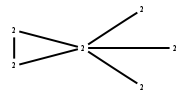# The Markov Bases Database

## G92g_bin

The binary graphical model of G92.

It is a hierarchical model of 6 variables. The dimension of the model is 13.The cardinality of the statespace is 64.

### Properties of the Markov basis

 Markov degree 2 516

 degree 2 516

The model has the following properties:

• All variables are binary.
• It is a graphical model.
• The semigroup is normal.### FILES

 Markov basis: sufficient statistics matrix: G92g_bin.mar (97.26 kb) G92g_bin.mat (2.53 kb) G92g_bin.mod (43 b) G92g_bin.tar.gz (7.35 kb)